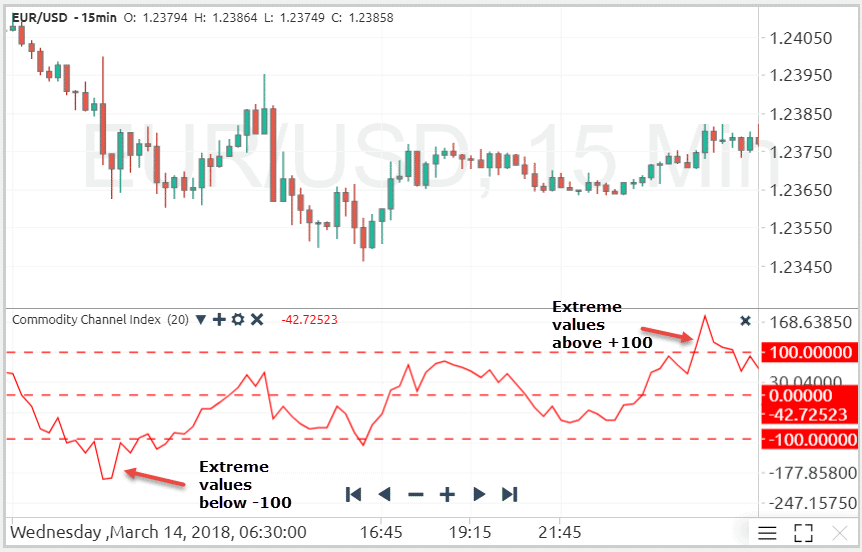# The Commodity Channel Index (CCI) ExplainedThe Commodity Channel Index (CCI) Explained
March 16, 2018

The Commodity Channel Index (CCI) indicator was developed by Donald Lambert in 1980s to spot trend reversals and extreme market conditions. Although the indicator was initially created to analyze the commodity market, it can be successfully applied to other financial markets as well.

The CCI indicator compares the current market price to an average price over a specified period of time. Basically, the indicator has a high reading if the current price is far above relative to the average price, and a low reading if the current price is far below relative to the average price.

## Calculation of the Commodity Channel Index

The CCI indicator uses the Typical Price (TP) in its calculation, which equals to the sum of the High, Low and Close prices divided by three. The 20-period simple moving average of the TP is then subtracted from the TP itself, to get the relative distance of the current market price from the average of the last 20 periods.

The final step includes the division of the result by (0.015 x Mean Deviation), in which 0.015 is a constant set by Lambert to ensure that around 75% of all CCI reading fall in the range between -100 and +100, and the Mean Deviation is calculated by three additional steps:

• Subtract the 20-period SMA from each period’s typical price
• Sum the absolute values of the results
• Divide the sum by the total number of periods used in the calculation (20).

Expressed as a formula, CCI is calculated as follows:

CCI = (Typical Price –  20-period MA of TP) / (0.015 x Mean Deviation)

Typical Price (TP) = (High + Low + Close)/3

A longer period-setting of the CCI caps more of the indicator’s values inside the range of -100 and +100, while a shorter period-setting creates a more volatile CCI with a lower percentage of values between -100 and +100.## How to Interpret the CCI

As the CCI represents the distance of the current period’s Typical Price from the average Typical Prices of the last n-periods, high indicator values indicate a strong bullish market, while low indicator values indicate a strong bearish market. The following chart shows the CCI indicator with a 20-periods setting, plotted on the EUR/USD pair.In this regard, if the CCI rises above +100 or below -100, traders can use the CCI to identify an emerging new trend or a reversal of the underlying trend. However, if traders try to trade overbought and oversold levels of the indicator, these two CCI strategies can return contrary trading signals.

This is the reason why you should first identify the current market environment, and adjust you trading strategy based on whether the market is trending or ranging.

In trending markets, a high CCI reading of above +100 could trigger a buy signal, while a CCI reading of below -100 would trigger a sell signal.

On the other hand, a range-bound market could be traded with a sell order once the indicator enters into overbought conditions, and with a buy order once the indicator enters into an oversold condition.

SummaryArticle Name
The Commodity Channel Index (CCI) Explained
Description
The Commodity Channel Index (CCI) indicator was developed by Donald Lambert in 1980s to spot trend reversals and extreme market conditions. Although the indicator was initially created to analyze the commodity market, it can be successfully applied to other financial markets as well.
Author
Publisher Name